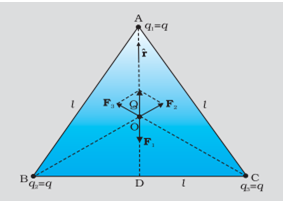Home/Class 12/Physics/

Consider three charges $$q_1,q_2,q_3$$ each equal to $$q$$ at the vertices of an equilateral triangle of side $$l$$ . What is the force on a charge $$Q$$ $$($$with the same sign as $$q)$$ placed at the centroid of the triangle, as shown in figure.Speed
00:00
03:21## QuestionPhysicsClass 12

Consider three charges $$q_1,q_2,q_3$$ each equal to $$q$$ at the vertices of an equilateral triangle of side $$l$$ . What is the force on a charge $$Q$$ $$($$with the same sign as $$q)$$ placed at the centroid of the triangle, as shown in figure.The equilateral triangle $$\mathit{ABC}$$  given, has equal length of each side $$l$$ , and if we draw a perpendicular line $$\mathit{AD}$$  to the side $$\mathit{BC}$$ , then
$$\mathit{AD}=\mathit{AC}\cos 30^0=(\sqrt 3/2)l$$
and the distance $$\mathit{AO}$$ of the centroid $$O$$  from $$A$$  is
$$(2/3$$
And by the law of symmetry, we know that $$\mathit{AO}=\mathit{BO}=\mathit{CO.}$$
Thus,
Force $$F_1$$ acting on $$Q$$  due to the charge $$q$$  at $$A=(3/4\pi \varepsilon _0)\times (\mathit{Qq}/l^2)$$ along dotted line $$\mathit{AO.}$$
Force $$F_2$$ acting on $$Q$$  due to the charge $$q$$  at $$B=(3/4\pi \varepsilon _0)\times (\mathit{Qq}/l^2)$$ along dotted line $$\mathit{BO.}$$
Force $$F_3$$ acting on $$Q$$  due to the charge $$q$$  at $$C=(3/4\pi \varepsilon _0)\times (\mathit{Qq}/l^2)$$ along dotted line $$\mathit{CO.}$$
Resultant of forces $$F_2$$ and $$F_3=(3/4\pi \varepsilon _0)\times (\mathit{Qq}/l^2)$$ along $$\mathit{OA}$$  by the parallelogram law.
Thus, the total force $$F$$ on charge $$Q$$ can be given as,
$$F=\frac 3{4\pi \varepsilon _0}\frac{\mathit{Qq}}{l^2}\left(r-r\right)=0$$
Where $$r$$ is the unit vector along dotted line $$\mathit{OA.}$$
Also it is revealed by symmetry that the three forces willobviously sum to zero.